# Populations, Samples, and Estimators¶

In this notebook we will examine the relationship between a population, a method for selecting a sample from the populations, and the resulting sample.

When we are in the situation where we have data from the population of interest, then we can directly examine the population distribution and parameters. In many situations we do not have the population and must work with a sample. Our goal in this case is to make inferences about the population. In this notebook, we will use the restaurant inspections as an example of a population and draw samples from the populations to figure out how we might use the sample for making inferences about the population.

In :
import os
from IPython.display import display, Latex, Markdown

In :
import pandas as pd
import numpy as np
import matplotlib.pyplot as plt
import seaborn as sns
%matplotlib inline
sns.set_context("talk")


## Restaurant Inspection Scores - The Population¶

Recall that we have an administrative data set that contains inspection scores for all restauarants in San Francisco over a three year period. Many restaurants have more than one inspection a year. If our population is restaurants, then we will want to focus on one set of scores. Let's choose one inspection score per restaurant from 2016.

Below, we read in the data, examine a few results, and create our population of restaurant inspection scores in 2016.

In :
dsDir = "data/"

ins = pd.read_csv("data/inspections.csv")

In :
ins.head(10)

Out:
business_id score date type
0 10 82 20160503 routine
1 10 94 20140729 routine
2 10 92 20140114 routine
3 19 94 20160513 routine
4 19 94 20141110 routine
5 19 94 20140214 routine
6 24 98 20161005 routine
7 24 96 20160311 routine
8 24 96 20141124 routine
9 24 96 20140612 routine
In :
ins.tail(10)

Out:
business_id score date type
15420 89328 84 20161206 routine
15421 89453 90 20161019 routine
15422 89462 76 20160929 routine
15423 89475 96 20161130 routine
15424 89495 100 20161008 routine
15425 89515 98 20161103 routine
15426 89701 100 20161206 routine
15427 89790 96 20161129 routine
15428 89803 100 20161215 routine
15429 89900 93 20161206 routine

We select one score per restaurant in 2016.

In :
ins['new_date'] = ins['date'].apply(lambda d: pd.datetime.strptime(str(d),'%Y%m%d'))
ins['year']     = ins['new_date'].apply(lambda d: d.year)

ins = ins[ins['year']==2016].groupby('business_id').last().copy()

In :
print("Number of Restaurants:", len(ins))
ins.head(10)

Number of Restaurants: 4543

Out:
score date type new_date year
business_id
10 82 20160503 routine 2016-05-03 2016
19 94 20160513 routine 2016-05-13 2016
24 96 20160311 routine 2016-03-11 2016
31 84 20160816 routine 2016-08-16 2016
45 78 20160104 routine 2016-01-04 2016
48 94 20160630 routine 2016-06-30 2016
50 80 20160614 routine 2016-06-14 2016
56 90 20160802 routine 2016-08-02 2016
58 73 20160407 routine 2016-04-07 2016
61 94 20160708 routine 2016-07-08 2016
In :
ins.tail(10)

Out:
score date type new_date year
business_id
89328 84 20161206 routine 2016-12-06 2016
89453 90 20161019 routine 2016-10-19 2016
89462 76 20160929 routine 2016-09-29 2016
89475 96 20161130 routine 2016-11-30 2016
89495 100 20161008 routine 2016-10-08 2016
89515 98 20161103 routine 2016-11-03 2016
89701 100 20161206 routine 2016-12-06 2016
89790 96 20161129 routine 2016-11-29 2016
89803 100 20161215 routine 2016-12-15 2016
89900 93 20161206 routine 2016-12-06 2016

### Population Distribution¶

Let's look at the distribution of inspections scores for the restaurant population.

In :
scoreCts = ins['score'].value_counts().sort_index()
scoreCts

Out:
52       1
55       3
56       2
57       6
58       1
59       4
60       3
61       5
62       4
63       7
64       2
65      10
66      13
67      17
68      26
69      14
70      23
71      29
72      35
73      26
74      42
75      37
76      34
77      46
78      61
79      58
80      70
81      92
82      89
83     126
84      96
85     121
86     183
87     160
88     190
89     124
90     315
91     109
92     395
93      77
94     403
96     524
98     304
100    656
Name: score, dtype: int64

Notice that scores are integer values and for this population they range between 52 and 100. There are no restaurants with a score of 95, 97, or 99. We make a bar plot (rather than a histogram) so that we can see all of the possible values for inspection score for the restaurants.

In :
plt.bar(scoreCts.index.values, scoreCts.values)
plt.xlabel('Restaurant score')
plt.ylabel('Frequency')
#plt.savefig("barplot_counts.pdf")
plt.show()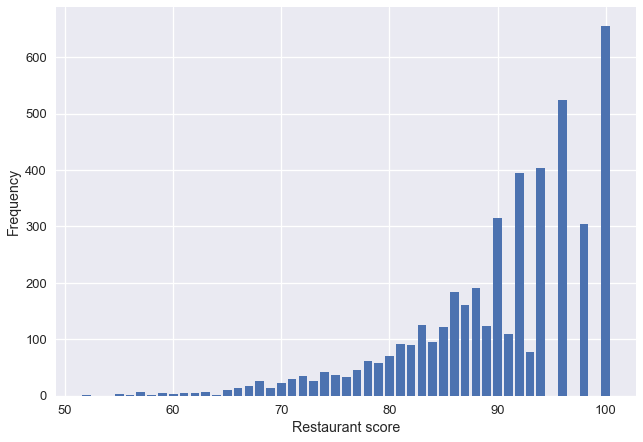Ideally, we want percentages on the y axis. This will it easier for us to compare the population distribution with the distribution of a sample and the probability distribution of a random draw from the population.

In :
100 * scoreCts/scoreCts.sum()

Out:
52      0.022012
55      0.066036
56      0.044024
57      0.132071
58      0.022012
59      0.088048
60      0.066036
61      0.110059
62      0.088048
63      0.154083
64      0.044024
65      0.220119
66      0.286155
67      0.374202
68      0.572309
69      0.308166
70      0.506273
71      0.638345
72      0.770416
73      0.572309
74      0.924499
75      0.814440
76      0.748404
77      1.012547
78      1.342725
79      1.276689
80      1.540832
81      2.025094
82      1.959058
83      2.773498
84      2.113141
85      2.663438
86      4.028175
87      3.521902
88      4.182258
89      2.729474
90      6.933744
91      2.399296
92      8.694695
93      1.694915
94      8.870790
96     11.534228
98      6.691613
100    14.439797
Name: score, dtype: float64

We could have normaized the counts to begin with as follows.

In :
scoreCts = 100 * ins['score'].value_counts(normalize = True).sort_index()
scoreCts

Out:
52      0.022012
55      0.066036
56      0.044024
57      0.132071
58      0.022012
59      0.088048
60      0.066036
61      0.110059
62      0.088048
63      0.154083
64      0.044024
65      0.220119
66      0.286155
67      0.374202
68      0.572309
69      0.308166
70      0.506273
71      0.638345
72      0.770416
73      0.572309
74      0.924499
75      0.814440
76      0.748404
77      1.012547
78      1.342725
79      1.276689
80      1.540832
81      2.025094
82      1.959058
83      2.773498
84      2.113141
85      2.663438
86      4.028175
87      3.521902
88      4.182258
89      2.729474
90      6.933744
91      2.399296
92      8.694695
93      1.694915
94      8.870790
96     11.534228
98      6.691613
100    14.439797
Name: score, dtype: float64
In :
plt.bar(scoreCts.index.values, scoreCts)
plt.xlabel('Restaurant score')
plt.ylabel('Percent')
#plt.savefig("barplot_percent.pdf")
plt.show()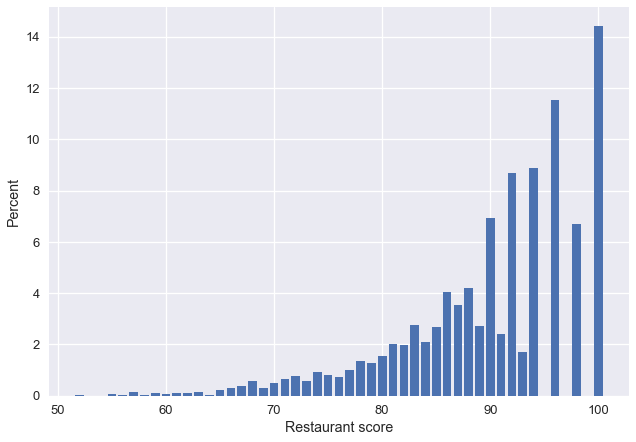### Population parameters - average and SD¶

We can examine simple summaries of the population distribution such as the average inspection score and the standard deviation of the inspection score.

Below we show that the sample average can be computed as a weighted average of the unique values in the population, where the weight for an inspection score is the proportion of restaurants with that score. That is,

$$\large \texttt{Population Average} = \frac{1}{N} \sum_{i=1}^N x_i,$$

where $x_i$ is the score for restaurant $i$, for $i=1, \ldots, N$.

Suppose there are $m$ unique values for the score, for $j = 1, \ldots, m$. Then, we can equivalently express the population average as

$$\large \texttt{Population Average} = \sum_{j=1}^m v_j \times p_j,$$

where $p_j$ is the proportion of the population with score $v_j$.

In :
print("The population average is: ", ins['score'].mean() )

print("Another way to compute this is as a weighted sum: ",
(scoreCts.index.values * scoreCts / 100).sum())

The population average is:  90.02927580893683
Another way to compute this is as a weighted sum:  90.02927580893682


The standard deviation (SD) of the scores is a measure of the typical deviations of an inspection score from the population mean. It is also called the root mean square error about the mean. This alternative name tells us how to compute the SD.

$$\large \texttt{Standard Deviation} = \sqrt{\sum_{i=1}^m \left(v_j - \texttt{Pop Avg} \right)^2 p_j}$$

Working from the inside outward, we see that we are examining the error about the mean (the loss), squaring it, taking the mean, and then the square root.

The std function performs this computation for us.

In :
print("The population SD is: ", ins['score'].std())

The population SD is:  8.333893943944487


The population of restaurants in 2016 in San Francisco have an average inspection score of 90 with a SD of 8.3.

## Probability Distribution of Draws from the population¶

In order to investigate the probability distribution of a draw from the population, we start with a much simpler example where the population is very small. This will make it easier for us to make probability calculations and generalize from these calculations to drawing from a more general population.

We set up a small population with 5 inspection scores: 80, 80, 92, 92, 96. As before, we can examine the population distribution, mean, and SD.

In :
scores_tiny = pd.Series([80, 80, 92, 92, 96], name="score")

scoreCts_tiny = scores_tiny.value_counts(sort = True)

In :
plt.bar(scoreCts_tiny.index.values, 100 * scoreCts_tiny/scoreCts_tiny.sum())
plt.xticks(np.arange(79, 101, 1))
#plt.savefig("barplot_percent_tiny.pdf")
plt.show()

print("The tiny population mean is: ", scores_tiny.mean())
print("The tiny population SD is: ", scores_tiny.std() )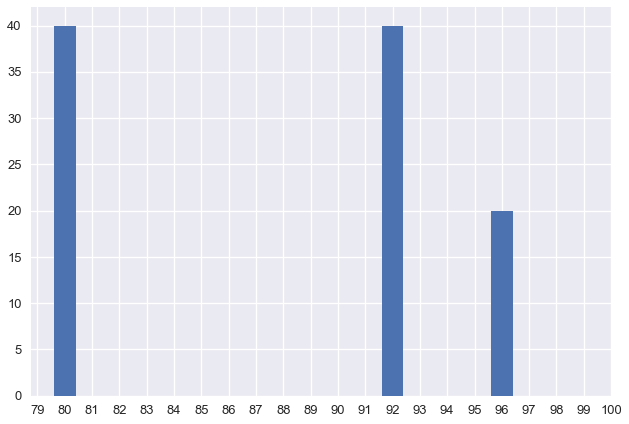The tiny population mean is:  88.0
The tiny population SD is:  7.483314773547883


### Draw one score at random¶

For this population, it is relatively easy for us to consider the probability distribution of one draw at random. When we say at random, we mean with equal chance.

If $X$ represents the possible outcome of this chance process, then $X$ can take on the value of 80 or 92 or 96. The probability distribution of $X$ summarizes the chance of each of these possible outcomes. We can summarize these probabilities in a table:

outcome 80 92 96
chance 2/5 2/5 1/5

### What do we expect?¶

What do we expect $X$ to be? To get a sense of this, we can use the computer to take a sample and repeat again and again to see how the results vary. We first repeat only 3 times to see how the values of $X$ vary.

In :
np.random.seed(37)
print("One sampled score", ins['score'].sample(1),
"\nAnother sampled score", ins['score'].sample(1),
"\nAnd another", ins['score'].sample(1))

One sampled score business_id
799    92
Name: score, dtype: int64
Another sampled score business_id
82803    94
Name: score, dtype: int64
And another business_id
9710    94
Name: score, dtype: int64


On the first draw, we got 92, on the secon 94 and the third is the same as the second.

Let's repeat this process one thousand times to see what the distribution of possible values for $X$ looks like.

In :
np.random.seed(77)
samples_tiny = pd.Series([scores_tiny.sample(1, replace = True).values
for _ in range(1000)])
samples_tiny.describe()

Out:
count    1000.000000
mean       88.336000
std         6.716861
min        80.000000
25%        80.000000
50%        92.000000
75%        92.000000
max        96.000000
dtype: float64

We see that the average value for the 1000 realizations of $X$ is 88.3. Recall that the population average is 88. We also see that the standard deviation of these 1000 realizations is 6.7. For comparison the SD of the population is 7.5.

This simulation that we performed gives us some insight as to the Expected Value of $X$ and the Standard Deviation of $X$.

Let's also examine the proportions of the 1000 values of $X$ that are 80, 92, and 96.

In :
scoreCts_samp = 100 * pd.value_counts(samples_tiny, normalize = True)

barSample = plt.bar(scoreCts_samp.index.values, scoreCts_samp.values)
plt.xticks(np.arange(80, 101, 2))
plt.yticks(np.arange(0, 45, 5))
plt.xlabel('1000 Sampled Restaurant Scores from Tiny Population (80, 80, 92, 92, 96)')
plt.ylabel('Percent')
plt.show()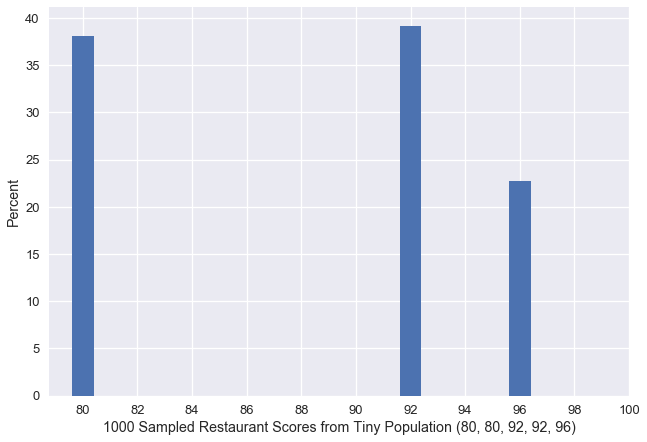Notice that the simulation produced proportions that are close to the probabilities. That is, in 1000 simulated draws from our tiny population, about 38% of the draws were 80. In comparison, the chance that $X$ is 80 is $2/5$ or 40%.

### From Simulation to Proof¶

The simulation gave us several insights:

• The probability distribution of $X$ matches the population distribution
• The expected value of $X$ is the population average.
• The SD of $X$ is the population SD.

Actually, we had figured the first of these discoveries out already. The last two of the discoveries, we can prove analytically. See the posted course notes.

Let's confirm that these discoveries hold for our larger population of all restaurants in San Francisco.

In :
np.random.seed(707)
samples = ins['score'].sample(1000, replace = True)
samples.describe()

Out:
count    1000.000000
mean       90.061000
std         8.190627
min        52.000000
25%        86.000000
50%        92.000000
75%        96.000000
max       100.000000
Name: score, dtype: float64

Recall that our population mean is 90 and SD is 8.3.

Let's examine the distribution of the 1000 simulated values of $X$ and compare this simulated distribution to the population distribution.

In :
scoreCts_samp = 100 * pd.value_counts(samples, normalize = True)

barSample = plt.bar(scoreCts_samp.index.values, scoreCts_samp.values)
plt.xticks(np.arange(50, 101, 10))
plt.yticks(np.arange(0, 15, 2))
plt.xlabel('1000 Sampled Restaurant Scores')
plt.ylabel('Percent')
#plt.savefig("barplot_samp1000.pdf")
plt.show()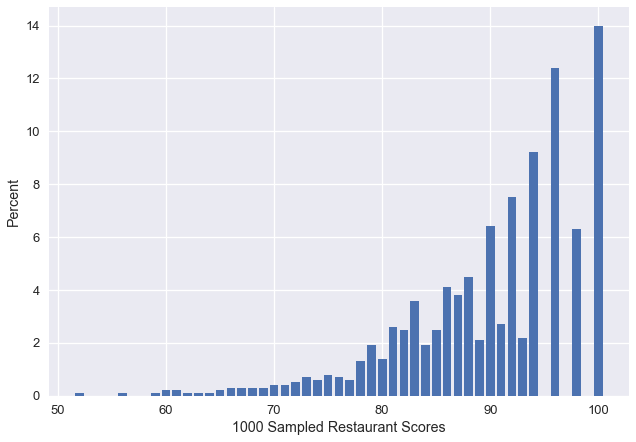In :
barPop = plt.bar(scoreCts.index.values, scoreCts.values)
plt.xticks(np.arange(50, 101, 10))
plt.yticks(np.arange(0, 15, 2))
plt.xlabel('Population of Restaurant Scores')
plt.ylabel('Percent')
#plt.savefig("barplot_pop.pdf")
plt.show()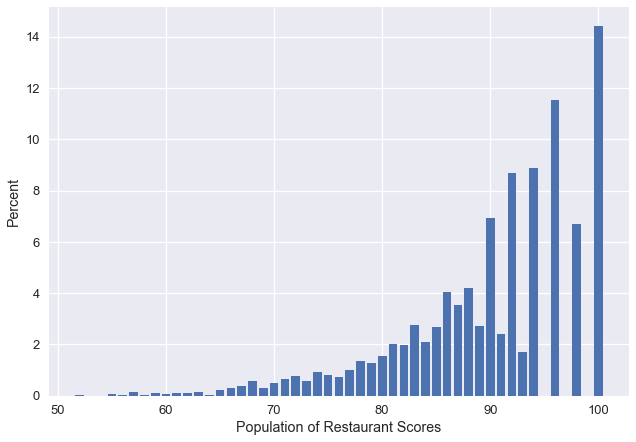Notice that in our sample of 1000 we did not observe all of the lowest values because these have low proportions in our population.

### Sampling 100 restaurants¶

Now suppose we take a sample of 100 restaurants at random without replacement.

What does any one sample look like?

In :
np.random.seed(107)
samples = ins['score'].sample(100, replace = False)
scoreCts_samp = pd.value_counts(samples, sort = True)

barSample = plt.bar(scoreCts_samp.keys(),
100 * scoreCts_samp.values/scoreCts_samp.values.sum())
plt.xticks(np.arange(50, 101, 10))
plt.yticks(np.arange(0, 15, 2))
plt.xlabel('Scores for Sample of 100 Restaurants')
plt.ylabel('Percent')
#plt.savefig("barplot_samp100.pdf")
plt.show()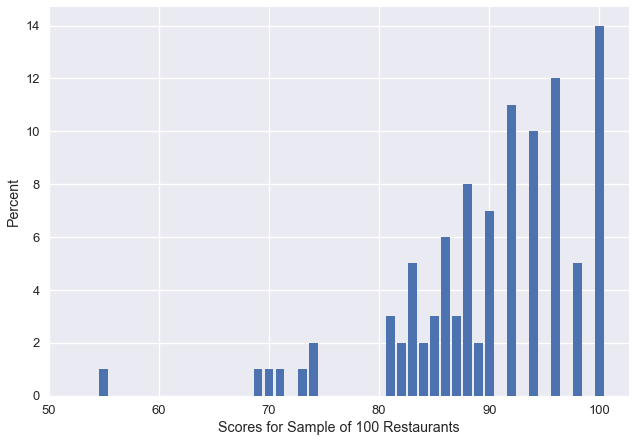In :
samples.mean()

Out:
90.14

The sample looks similar to the population distribution, although it's clearly different.

Consider the 100 random variables, $X_1$, $X_2$, ... $X_{100}$ that result from 100 draws at random without replacement from the population. Each of these random variables has the same distribution. This implies that $$\large \textbf{E}(X_i) = \texttt{population average}$$ In turn, this then implies that $$\large \textbf{E}(\bar{X}) = \texttt{population average}$$ (See the probability handout for a proof).

Since the $X_i$ have the same distribution, we also find that $$\large SD(X_i) = \textrm{population SD} = \sigma$$ However,
$$\large SD(\bar{X}) = \frac{N-n}{N-1}\frac{\sigma}{\sqrt{n}},$$ rather than $\frac{\sigma}{\sqrt{n}}$ because the $X_i$ are dependent random variables.

We can use simulation studies to develop insight into these results and others.

### Simulating the Probability Distribution of $\bar{X}$¶

What does the probabiity distribution of the sample average look like?

We can simulate this distribution by taking 1000 replications: where for each replicate we take a simple random sample of 100 values from the population and find the sample average. Repeating this 1000 times gives us an idea as to what the sampling distribution of the average looks like.

In :
np.random.seed(707)
res = pd.Series([
ins['score'].sample(100, replace = False).mean()
for _ in range(1000)
])
res.describe()

Out:
count    1000.000000
mean       90.041820
std         0.809807
min        87.100000
25%        89.517500
50%        90.070000
75%        90.552500
max        92.740000
dtype: float64
In :
sns.distplot(res)
#plt.savefig('mean_hist1000v2.pdf')

Out:
<matplotlib.axes._subplots.AxesSubplot at 0x115064cf8>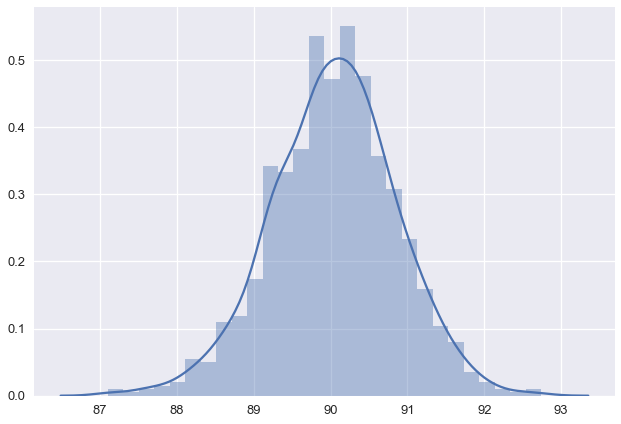As noted earlier, the simulation indicates that expected value of $\bar{X}$ should match the population average, which is 90. We can also see that the SD of $\bar{X}$ is about $(4443/4542) \times \sigma/\sqrt{100} \approx 0.82$. When we compute an SD of a statistics, we usually called is the Standard Error or SE for short.

Notice that we have discovered via simulation that the probability distribution of the sample average roughly looks like the normal curve. It is unimodal, symmetric, with neither long nor short tails.

## Monte Carlo¶

We will carry out some more detailed simulation studies to examine the expected value, standard deviation, and distribution of the sample average for averages of different sizes. In the process we will confirm our theoretical results and discover new ones.

These are examples of a simple but powerful approach to studying random processes through simulation. The technique that we have been using is called Monte Carlo simulation. Briefly, suppose that we want to study the behavior of a statistic $T(X_1, \ldots, X_n)$ which is a function of $X_1, \ldots X_n$. (The sample mean, median, Huber estimator are all examples). Then we can study the behavior of $T$ under different conditions, such as a rango of sample sizes, different population distributions, sampling with or without replacement, etc. We perform the Monte Carlo as follows:

• Take a combination of input values, e.g., sample size and population distribution, which we call a data generation model
• Generate data from this model and calculate the statistic of interest
• Repeat this process many (typically thousands) of times
• Repeat the above process for all combinations of input values (data generation models).

We will study the impact of the sample size on the probability distribution of $\bar{X}$ for the restaurant population and sampling with replacement.

### Law of Large Numbers - demonstration¶

We begin by setting up a range of sample sizes that we want to study.

In :
sample_sizes = np.exp(np.linspace(np.log(10), np.log(10000), 50)).astype("int")

sample_sizes

Out:
array([   10,    11,    13,    15,    17,    20,    23,    26,    30,
35,    40,    47,    54,    62,    71,    82,    95,   109,
126,   145,   167,   193,   222,   255,   294,   339,   390,
449,   517,   596,   686,   790,   910,  1048,  1206,  1389,
1599,  1842,  2120,  2442,  2811,  3237,  3727,  4291,  4941,
5689,  6551,  7543,  8685, 10000])

Next we carry out one round of our Monte Carlo study. That is, we examine the sample average for all of our sample sizes, where we generate only one sample for each size.

In :
np.random.seed(707)
plt.figure(figsize=(15,10))

pd.Series([
ins['score'].sample(s, replace = True).mean()
for s in sample_sizes
], index=sample_sizes).plot()
plt.yticks(np.arange(86, 95, 2))
plt.xscale('log')
plt.xlabel('Sample Size (log scale)')
plt.ylabel('Average Inspection Score')
#plt.savefig('lln.pdf')

Out:
<matplotlib.text.Text at 0x11807f048>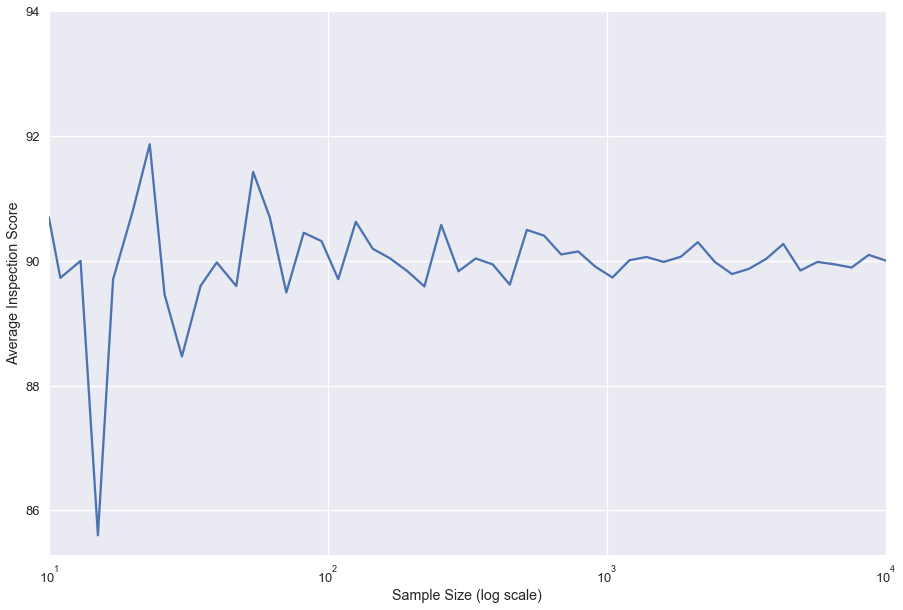It appears that the sample average gets closer to the population average as the sample size grows. Note that the x-axis is on log scale.

We repeat this process 100 times to get a sense of how quickly the sample average converges to the population mean. Not that we use transparency to help see where are the bulk of values.

In :
plt.figure(figsize=(15,10))

for i in range(0,100):
pd.Series([
ins['score'].sample(s, replace = True).mean()
for s in sample_sizes
], index=sample_sizes).plot(color='grey', alpha = 0.2)

plt.yticks(np.arange(86, 95, 2))
plt.xscale('log')
plt.xlabel('Sample Size (log scale)')
plt.ylabel('Average Inspection Score')
#plt.savefig('lln_many.pdf')

Out:
<matplotlib.text.Text at 0x117d65e10>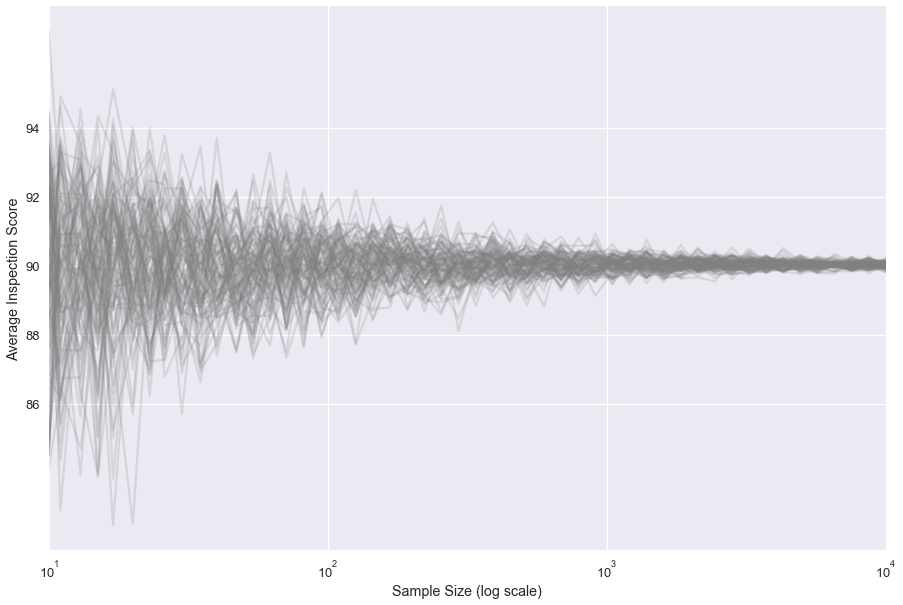We have discovered the Law of Large Numbers: As the sample size increases, the sample average (from indpendent sample with replacement from a population) converges to the population average.

### Square-root law¶

We already know the Square-root Law: The standard error of the sample average shrinks by a facotr of $1/\sqrt{n}$ with the sample size. However, we can confirm this is the case with a simulation study. Again, we vary the sample size. This time we take 1000 replications for each sample size. That is for a particular sample size, we obtain 1000 sample averages, and we find the standard deviation of these 1000 sample averages to approximate the SD of the sample average.

In :
sample_sizes = np.exp(np.linspace(np.log(10), np.log(10000), 50)).astype("int")

sds = []

for s in sample_sizes:
means = [
ins['score'].sample(s, replace = True).mean()
for _ in range(1000)]
sds.append(np.std(means))


Since we want to confirm the Square-root Law, we plot our findings along with our theory, i.e., $\sigma/\sqrt{n}$. The two curves are essentially on top of each other.

In :
plt.figure(figsize=(10,7))

plt.plot(sample_sizes, sds, label='SE for 1000 Simulated Means')
plt.plot(sample_sizes, ins['score'].std()/np.sqrt(sample_sizes),
label='Population SD/sqrt(sample size)')

plt.xscale('log')
plt.xlabel('Sample Size (log scale)')
plt.ylabel('Standard Error of Mean')
plt.legend()
#plt.savefig('sqrt_law.pdf')

Out:
<matplotlib.legend.Legend at 0x118dabe10>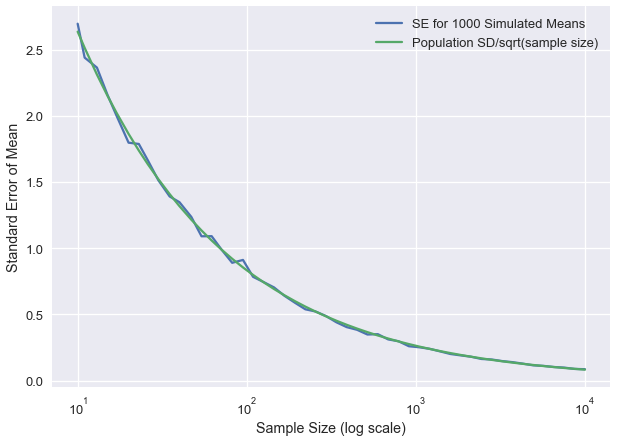### Central Limit Theorem Demonstration¶

We will discover one more theoretical result via simulation. Note that when we plotted the simulated sampling distribution of the average for 100 samples, we saw that the histogram appeared to roughly follow the normal curve. Let's see how the sampling distribution depends on the sample size. We consider 5 samples sizes: 20, 40, 100, 400, 1000.

In :
sample_sizes = [20, 40, 100, 400, 1000]
data = {}
for s in sample_sizes:
means = [
ins['score'].sample(s, replace = True).mean()
for _ in range(10000)]
data[s] = means

In :
plt.figure(figsize=(10,7))

for s in sample_sizes:
sns.distplot(data[s], label=str(s))
plt.legend()
#plt.savefig("histogram.pdf")

Out:
<matplotlib.legend.Legend at 0x118dbba90>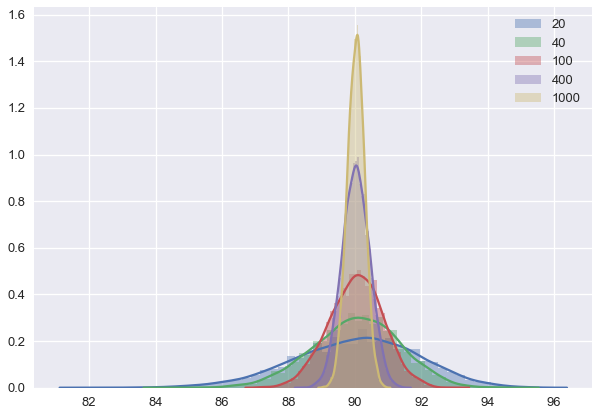We have discovered that as the sample size grows, the distribution appears to follow the normal curve more closely. Additionally, the center of the distribution is at the population mean and the spread of the distribution shrinks (it depends on the SD of the distribution which we know decreases like $1/\sqrt{n}$.

This is the Central Limit Theorem For $n$ large in absolute terms and small relative to $N$ (if sampling without replacement), the probability distribution of the sample average becomes increasingly close to the normal curve with center at the population average and SD $= \sigma/\sqrt{n}$.

## Restaurant Inspection Scores - The Sample¶

Now suppose we are in the realm where we do not know or see the population and we have one sample of 100.

Suppose that we want to use our sample to make statements about the population.

Here is out sample:

In :
np.random.seed(37)
my_sample = ins['score'].sample(100, replace = False)
scoreCts_samp = 100 * pd.value_counts(my_sample, normalize = True)

barSample = plt.bar(scoreCts_samp.index.values,
scoreCts_samp.values)
plt.xticks(np.arange(50, 101, 10))
plt.yticks(np.arange(0, 15, 2))
plt.xlabel('Scores for Sample of 100 Restaurants')
plt.ylabel('Percent')
#plt.savefig("barplot_mysamp.pdf")
plt.show()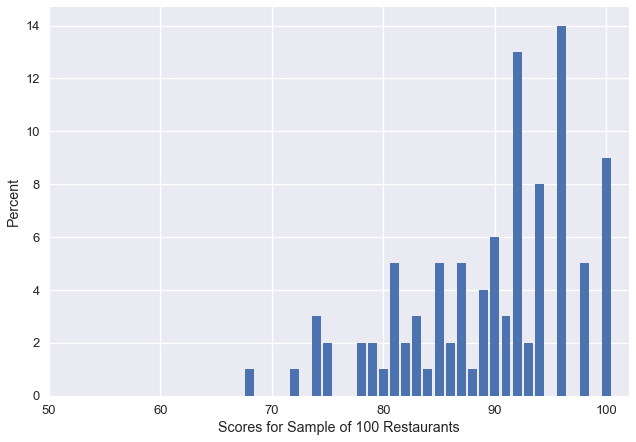We have seen in our earlier simulation studies that the Empirical Distribution of the sample looks approximately like the Population Distribution. This implies that parameter estimates based on our sample should be relatively good estimates of our population parameters.

In fact, the theory that we have proven or discovered via simulation that:

• the expected value of the sample average is the population average
• the variability in the sample average decreases like $\sigma/\sqrt{n}$
• for $n$ large, the sample average behaves like one draw from a distribution that looks like the normal curve that is centered at the population average and has a spread of $\sigma/\sqrt{n}$

We can use these results to make statements about the population average. For example, we can say that the population average is near:

In :
np.mean(my_sample)

Out:
89.65

If we approximate the population SD with the sample SD (why is that OK), we can say that we expect the error of our estimate to be about:

In :
np.std(my_sample)/np.sqrt(len(my_sample))

Out:
0.7412658092749187

### Bootstrapping the Sample Average¶

What happens when we want to estimate a parameter other than the population mean, such as a population percentile? The theory that we have derived and simulated for the mean is a bit more delicate for other estimators. For example, we may not be able to use the $\texttt{SD}(sample)/\sqrt{n}$ as an approximation for the SE of the estimaotr. Indeed, if the sampling distribution of the estimator is not close to normal, we may want to use a different approach for providing an estimate of the variabiity in our estimator?

In this situation, we fall back on the mechanism for generating the data: the Simple Random Sample (whether with or without replacement). We have seen the empirical distribution of the sample is similar to the population distribution. We use this similarity to bootstrap ourselves into the realm of a population. In particular, we use the sample proportions as the population distribution. This is called the bootstrap population. From there we essentially perform Monte Carlo simulation with this population to understand the behavior of the paramter estimator.

That is, we take a sample from the bootstrap population, which we call a bootstrap sample. For the bootstrap sample, we calculate the estimator (e.g., mean, percentile, Huber estimator), which we call the bootstrap statistic. We repeat this process thousands of times and study the distribution of the bootstrap sampling distribution.

Note that it is the shape and variability of the bootstrap sampling distribution that is of interest to us. The average is not of interest because by design, it will align with the bootstrap population (our original sample), not the true population.

The code is nearly the same as before, we simply draw at random from our sample, rather than from the population. For now, we will ignore the difference between sampling with and without replacement and assume that we have a large enough population size that these are essentially equivalent.

In :
np.random.seed(37)
boot_means = [
my_sample.sample(100, replace = True).mean()
for _ in range(10000)]
boot_means[0:20]

Out:
[89.7,
89.01,
89.72,
89.0,
89.89,
90.81,
89.66,
89.1,
88.8,
89.25,
89.79,
89.58,
89.33,
89.38,
91.5,
89.79,
90.27,
89.42,
89.24,
90.25]
In :
sns.distplot(boot_means)
plt.xlabel('10000 Bootstrapped Sample Averages (sample size = 100)')
#plt.savefig("bootstrap_hist.pdf")

Out:
<matplotlib.text.Text at 0x119b1afd0>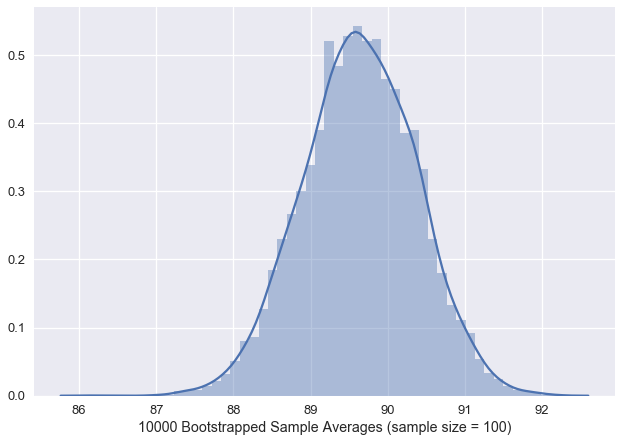As mentioned, the average of the 10000 bootstrapped means is very close to our sample average, 89.65, not the population average. This again is because the bootstrap population has a mean of 89.65 and that is the population that we are sampling from.

In :
np.mean(boot_means)

Out:
89.634312999999992

Also notice that the SD of the 10000 sample averages is close to our sample average/10, i.e., 0.74, rather than the population average/10, which is 0.83. Given the skewness of the population (and the sample) there is another version of the bootstrap that is typically better to use in this situation. It is called the standardized bootstrap. We may introduce it.

Nonetheless, this is a rough approximation to the standard error of the statistic of interest.

In :
np.std(boot_means)

Out:
0.73520482046229807

We will consider the bootstrap for other statistics next.